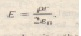# Applying Gauss’ law Cylindrical Symmetry

Applying Gauss’ law Cylindrical Symmetry

The drum of the photocopying machine in Exercise  has a length of 42 cm and a diameter of 12 cm What is the total charge on the drum (b) The manufacturer wishes to produce a desktop version of the machine This require reducing the size of the drum to a length of 28 cm and a diameter of 8.0 cm. The electric field at the drum surface must remain unchanged. What must be the charge on this new drum An infinite line of charge produces a field of 4.5 x 104 N/C at a distance of 2.0 m. Calculate the linear charge densite shows a section of a long. them-walled metal tube of radius R. with a charge per unit length A on its surface. Deriv c expressions
for E in terms of the distance r from the tube axis considering both (a) r > Hand (b) r < R. Plot your results for the range r = 0 to r = 5.0 em.
assuming that A = 2.0 X JO ” C/m and R = 3.0 Col. (HI/II: Use C) cylindrical Gaussian surfaces. convivial with the mew I tub  A very long conducing C)- cylindrical rod of length L a  charge +q is surrounded by a conducting cylindrical shell charge   Use  to  (a) the electric field at points outside the
conducting shell (11) the distribution of charge on the shell and (c) the electric field in the region between the shell and rod  A long straight wire has fixed negative charge with a linear charge density of magnitude The wire ;5 to be enclosed by a thin. nonconducting cylinder of outside radius
coaxial with the wire The cylinder is to has e positive charge on its outside surface” ith a surface charge density a such that the net external electric field is zero. Calculate the required  Two long charged concentric cylinders have radii of 3.0 and 6.0 Col. The charge per unit length is 5.0 x 10 6 C/m on the inner cylinder and -7.0 X 10 6 C/m on the outer cylinder. Find the